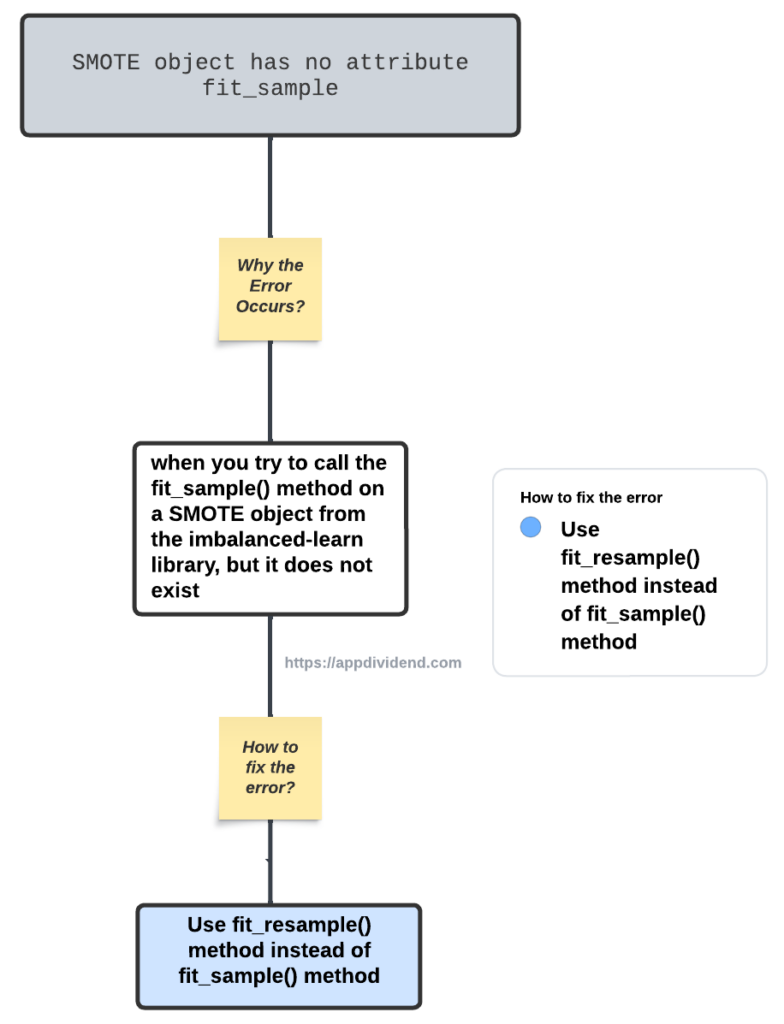# How to Fix AttributeError: ‘SMOTE’ object has no attribute ‘fit_sample’Diagram

AttributeError: ‘SMOTE’ object has no attribute ‘fit_sample’ error occurs when you “try to call the fit_sample() method on a SMOTE object from the imbalanced-learn library, but it does not exist.”

To fix the AttributeError: ‘SMOTE’ object has no attribute ‘fit_sample’ error, use the “fit_resample()” method instead of “fit_sample()” method.

## Reproduce the error

``````from imblearn.over_sampling import SMOTE
from sklearn.datasets import make_classification

# Create a sample imbalanced dataset
X, y = make_classification(n_classes=2, class_sep=2,
weights=[0.1, 0.9], n_informative=3, n_redundant=0,
flip_y=0, n_features=5, n_clusters_per_class=1,
n_samples=1000, random_state=42)

# Create a SMOTE object
smote = SMOTE(random_state=42)

# Fit and resample the dataset using SMOTE
X_resampled, y_resampled = smote.fit_sample(X, y)``````

Output

``````AttributeError: 'SMOTE' object has no attribute 'fit_sample'
``````

## How to fix it?

Replace the fit_sample() call with the fit_resample() method, which should resolve the issue.

``````from imblearn.over_sampling import SMOTE
from sklearn.datasets import make_classification

# Create a sample imbalanced dataset
X, y = make_classification(n_classes=2, class_sep=2,
weights=[0.1, 0.9], n_informative=3, n_redundant=0,
flip_y=0, n_features=5, n_clusters_per_class=1,
n_samples=1000, random_state=42)

# Create a SMOTE object
smote = SMOTE(random_state=42)

# Fit and resample the dataset using SMOTE
X_resampled, y_resampled = smote.fit_resample(X, y)
``````

If you run the above code, it won’t give you any error!

## Related posts

AttributeError: ‘RandomUnderSampler’ object has no attribute ‘fit_resample’

AttributeError: ‘Simple_Imputer’ object has no attribute ‘fill_value_categorical’

AttributeError: ‘Pipeline’ object has no attribute ‘fit_resample’

This site uses Akismet to reduce spam. Learn how your comment data is processed.